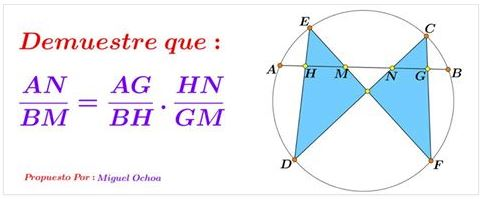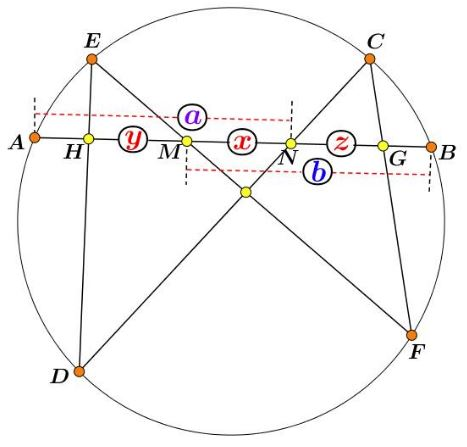# Generalization of Butterfly's Theorem

### Miguel Ochoa Sanchez 28 February, 2017#### Part 1#### Part 2### Remark

Assuming $a=b,\,$ the main result becomes $(a+z)(y+x)=(b+y)(z+x)\,$ which reduces to $(a-x)(y-z)=0,\,$ and, since $a\ne x,\,$ $y=z,\,$ proving the Butterfly theorem.### Butterfly Theorem and Variants